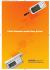# Mesh Layouts for Block-Based Caches

## Transcription

Mesh Layouts for Block-Based Caches
```IEEE TRANSACTIONS ON VISUALIZATION AND COMPUTER GRAPHICS, VOL. 12, NO. 5, SEPTEMBER/OCTOBER 2006
Mesh Layouts for Block-Based Caches
Sung-Eui Yoon, Member, IEEE, and Peter Lindstrom, Member, IEEE
Abstract—Current computer architectures employ caching to improve the performance of a wide variety of applications. One of
the main characteristics of such cache schemes is the use of block fetching whenever an uncached data element is accessed. To
maximize the benefit of the block fetching mechanism, we present novel cache-aware and cache-oblivious layouts of surface and
volume meshes that improve the performance of interactive visualization and geometric processing algorithms. Based on a general
I/O model, we derive new cache-aware and cache-oblivious metrics that have high correlations with the number of cache misses
when accessing a mesh. In addition to guiding the layout process, our metrics can be used to quantify the quality of a layout, e.g.
for comparing different layouts of the same mesh and for determining whether a given layout is amenable to significant improvement.
We show that layouts of unstructured meshes optimized for our metrics result in improvements over conventional layouts in the
performance of visualization applications such as isosurface extraction and view-dependent rendering. Moreover, we improve upon
recent cache-oblivious mesh layouts in terms of performance, applicability, and accuracy.
Index Terms—Mesh and graph layouts, cache-aware and cache-oblivious layouts, metrics for cache coherence, data locality.
F
1
I NTRODUCTION
Many geometric algorithms utilize the computational power of CPUs
and GPUs for interactive visualization and other tasks. A major trend
over the last few decades has been the widening gap between processor speed and main memory access speed. As a result, system architectures increasingly use caches and memory hierarchies to avoid
memory latency. The access times of different levels of a memory
hierarchy typically vary by orders of magnitude. In some cases, the
running time of a program is as much a function of its cache access
pattern and efficiency as it is of operation count .
One of the main characteristics of memory hierarchies is the use of
block fetching whenever there is a cache miss. Block fetching schemes
assume that there is high spatial coherence of data accesses that allow
repeated cache hits. Therefore, to maximize the benefit of block fetching, it is important to organize and access the data in a cache-coherent
manner. There are two standard techniques for minimizing the number
of cache misses: computation reordering and data layout optimization.
Computation reordering is performed to improve data access locality,
e.g. using compiler optimizations or application specific hand-tuning.
On the other hand, data layout optimization reorders the data in memory so that its layout matches the expected access pattern. In this paper,
we focus on computing cache-coherent layouts of polygonal and polyhedral meshes, in which vertices and cells (e.g. triangles, tetrahedra)
are organized as linear sequences of elements.
Many layouts and representations (triangle strips , space-filling
curves , stream  and cache-oblivious  layouts) have been
proposed for cache-coherent access. However, previous layouts have
either been specialized for a particular cache [5, 13] or application [14, 17], including graph and sparse matrix computations , or
are constructive [3,7,8,20,23,30,31] with no measure of global layout
quality needed to establish optimality, relative ranking among layouts,
and criterion for driving more general optimization strategies. Furthermore, while prior metrics may be suitable for their intended applications, they are not particularly good estimators of layout quality in the
context of block-based caching.
Main Results: We propose novel metrics and methods to evaluate and optimize the locality of mesh layouts. Based on a general I/O
model, we derive cache-aware and cache-oblivious metrics that corre• The authors are with the Lawrence Livermore National Laboratory,
E-mail: {sungeui, pl}@llnl.gov.
Manuscript received 31 March 2006; accepted 1 August 2006; posted online 6
November 2006.
[email protected]
(a) row-by-row
a: 8.50, g: 4.00, c: 150
(b) MLA 
a: 7.67, g: 3.45, c: 114
(c) Z curve 
a: 8.50, g: 3.35, c: 105
(d) H curve 
a: 13.15, g: 2.87, c: 102
(e) Hilbert curve 
a: 9.92, g: 2.73, c: 100
(f) β Ω curve 
a: 9.88, g: 2.70, c: 95
Fig. 1. Layouts and Coherence Measures for a 16×16 Grid: a and g correspond
to the arithmetic and geometric mean index difference of adjacent vertices; c denotes the cut, or number of edges that straddle cache blocks. Each block except
the last contains 27 vertices. MLA is known to minimize a, and β Ω is near-optimal
with respect to g for grids. Our new cache-oblivious measure, g, correlates better
than a with the cut and, hence, the number of cache misses.
late well with the number of cache misses when accessing a mesh in a
reasonably coherent, though not specific manner. Using these metrics,
we employ a multi-level recursive optimization method to efficiently
compute cache-coherent layouts of massive meshes consisting of hundreds of millions of vertices. We also observe that recursively constructed layouts, regardless of additional ordering criteria, in general
have good locality according to our metric.
Benefits: Our approach offers the following advantages over the
current state of the art:
• Generality: Our algorithm is applicable to any data set whose
expected access pattern can be expressed in a graph structure.
• Simplicity: Our metrics are concise and easy to implement.
• Accuracy: Our derived metrics correlate well with the observed
number of runtime cache misses. Hence our metrics are useful
for constructing good layouts.
• Efficiency: Our metrics can quickly quantify the quality of a
given layout. If according to the metric a layout already is coher-
IEEE TRANSACTIONS ON VISUALIZATION AND COMPUTER GRAPHICS, VOL. 12, NO. 5, SEPTEMBER/OCTOBER 2006
ent, no further work is needed to reorganize it, which saves time
and effort when dealing with very large meshes.
• Performance: Computed layouts optimized for our metrics
show performance improvements over other layouts.
We apply our cache-coherent layouts in two applications: isosurface extraction from tetrahedral meshes and view-dependent rendering of polygonal meshes. In order to illustrate the generality of our
approach, we compute layouts of several kinds of geometric models,
including a CAD environment, scanned models, an isosurface, and a
tetrahedral mesh. We use these layouts directly without having to modify the runtime application. Our layouts reduce the number of cache
misses and improve the overall performance.
2 R ELATED W ORK
Cache-efficient algorithms have received considerable attention over
last two decades in theoretical computer science and in the compiler
literature. These algorithms include models of cache behavior 
and compiler optimizations based on tiling, strip-mining, and loop
interchanging; all of these algorithms have shown to reduce cache
misses . Cache-efficient algorithms can be classified as computation reordering and data layout optimization.
2.1 Computation Reordering
Computation reordering strives to achieve a cache-coherent order of
runtime operations in order to improve program locality and reduce the
number of cache misses. This is typically performed using compiler
optimizations or application-specific hand tuning.
At a high level, computation reordering methods can be classified as either cache-aware or cache-oblivious. Cache-aware algorithms utilize knowledge of cache parameters, such as cache block
size . On the other hand, cache-oblivious algorithms do not assume any knowledge of cache parameters . There is a considerable amount of literature on developing cache-efficient computation
reordering algorithms for specific problems and applications, including numerical programs, sorting, geometric computations, matrix multiplication, FFT, and graph algorithms. More details are given in recent
surveys [2,28]. In visualization and computer graphics, out-of-core algorithms are designed to handle massive models using finite memory,
typically by limiting access to a small, cached subset of a model. A recent survey of these algorithms and their applications is given in .
2.2 Data Layout Optimization
The order in which data elements are stored can have a major impact on runtime performance. Therefore, there have been considerable
efforts on computing cache-coherent layouts of the data to match its
anticipated access pattern. The following possibilities have been considered.
Graph and Matrix Layouts: Graph and matrix layout problems
fall in the class of combinatorial optimization problems. Their main
goal is to find a linear layout of a graph or matrix that minimizes a
specific objective function. Well known minimization problems include linear arrangement (sum of edge lengths, i.e. index differences
of adjacent vertices), bandwidth (maximum edge length), profile (sum
of maximum per-vertex edge length), and wavefront (maximum front
size; see also ). This work has been widely studied and an extensive survey is available . While potentially improving data coherence, these layouts are not optimal—or necessarily good—for blockbased caching, as we shall see later.
Rendering Sequences: Modern GPUs maintain a small buffer
to reuse recently accessed vertices. In order to maximize the benefits
of vertex buffers for fast rendering, triangle reordering is necessary.
This approach was pioneered by Deering . The resulting ordering of
triangles is called a triangle strip or a rendering sequence. Hoppe 
casts the triangle reordering as a discrete optimization problem with
a cost function dependent on a specific vertex buffer size. Several
techniques improve the rendering performance of view-dependent algorithms by computing rendering sequences not tailored to a particular
cache size [3, 7, 17, 30].
Processing Sequences: Isenburg et al.  proposed processing sequences as an extension of rendering sequences to large-data
processing. A processing sequence represents an indexed mesh as
interleaved triangles and vertices that can be streamed through main
memory . Global mesh access is restricted to a fixed traversal order; only localized random access to the buffered part of the mesh is
supported as it streams through memory. This representation is mostly
useful for offline applications (e.g., simplification and compression)
that can adapt their computations to the fixed ordering.
Space-Filling Curves: Many algorithms use space-filling curves
 to compute cache-friendly layouts of volumetric grids or height
fields. These layouts are widely used to improve the performance of
image processing  and terrain or volume visualization [20, 23]. A
standard method of constructing a mesh layout based on space-filling
curves is to embed the mesh or geometric object in a uniform structure
that contains the space-filling curve. Gotsman and Lindenbaum investigated the spatial locality of space-filling curves . Motivated by
searching and sorting applications, Wierum  proposed using a logarithmic measure of edge length, resembling one of our new metrics,
for analyzing space-filling curve layouts of regular grids. Our results,
however, indicate that space-filling curve embedding does not work
well for meshes that have an irregular distribution of geometric primitives. Recently, Yoon et al. proposed methods for cache-coherent layout of polygonal meshes  and bounding volume hierarchies .
These methods are constructive in nature and require making a sequence of binary decisions without relying on a global measure of
locality. Hence, these methods depend on a particular optimization
framework and cannot be used to judge the quality of a layout.
3 C OMPUTATION M ODELS
In this section we describe an I/O model for our cache representation.
We also propose both a graph representation and probability model to
capture the likelihood of accessing mesh elements.
3.1 I/O Model
Most modern computers use hierarchies of memory levels, where each
level of memory serves as a cache for the next level. Memory hierarchies have two main characteristics. First, lower levels are larger
in size and farther from the processor, and hence have slower access
times. Second, data is moved in blocks of many elements between
different memory levels. Data is initially stored in the lowest memory level, typically the disk. A transfer is performed whenever there
is a cache miss between two adjacent levels of the memory hierarchy.
Due to this block fetching mechanism, cache misses can be reduced by
storing in the same block data elements that are accessed together.
We use a simple two-level I/O-model defined by Aggarwal and Vitter  that captures the two main characteristics of a memory hierarchy. This model assumes a fast memory called “cache” consisting
of M blocks and a slower infinite memory. The size of each cache
block is B; therefore, the total cache size is M × B. Data is transferred
between the levels in blocks of consecutive elements.
3.2 Graph Representation
Our layout algorithm requires the specification of a directed graph that
represents the anticipated runtime access pattern, and in which each
node represents a data element (e.g., mesh vertex or triangle). A directed arc (i, j) between two nodes indicates an expectation that node
j may be accessed immediately after node i. We chose directed graphs
to represent access patterns, in contrast to the undirected graphs used
in Yoon et al. , as we do not necessarily assume symmetry in node
access patterns.
Let G = (N, A) be a directed graph, where N is a set of nodes and A
is a set of directed arcs. The layout problem reduces to computing the
one-to-one mapping ϕ : N → {1, ..., |N|} of nodes to positions in the
layout that minimizes the expected number of cache misses.
We also require probabilities (as weights) for each node and arc that
represent the likelihood of accessing them at runtime. We derive these
probabilities here based on the graph structure by considering an infinite random walk over the graph. For each directed arc, (i, j) ∈ A,
YOON et al.: MESH LAYOUTS FOR BLOCK-BASED CACHES
let Pr( j|i) denote the probability of accessing node j next, given that
i was the previously accessed node. We define P to be a probability
transition matrix whose (i, j)th element equals Pr( j|i). Furthermore,
let Pr(i) denote the probability of accessing node i among all nodes
N, and let x be the n-by-1 column vector whose ith component equals
Pr(i). Given an initial state of node access probabilities, we update
each node’s probability in x by traversing the node’s outgoing arcs,
each with probability of access Pr( j|i). This update is equivalent to
premultiplying x by PT . If applied repeatedly, x will ultimately converge to a stable equilibrium
Layout algorithm: Constructing a layout optimized for
ECM1B (ϕ ) reduces to a k-way graph partitioning problem. Each
1
, and we partition the input
directed arc has a constant weight, |A|
n
graph into k = d B e different sets of vertices. Since graph partitioning
is an NP-hard problem, we rely on heuristics. One good heuristic
is the multi-level algorithm implemented in the METIS library .
Once the directed graph is partitioned, the ordering among blocks and
the order of vertices within each block do not matter in our I/O model.
x = PT x
We now assume that the cache holds multiple blocks. As in the singleblock case, a cache miss can occur only when we traverse an arc, (i, j),
that crosses a block boundary, i.e., ϕ B (i) = Bi 6= B j = ϕ B ( j). However, unlike the single-block case, block B j may already be stored in
the cache when we access j. Therefore, to compute the expected number of cache misses for a multi-block cache, we must also consider the
probability, Prcached (B j ), that B j is cached among the M blocks.
In theory, Prcached (B j ) can be computed by exhaustively generating
all possible access patterns for which B( j) is already cached when
we access j from i. Such block access patterns take on the form
(B j , . . . , Bi , B j ), where at most M different blocks are accessed before
B j is accessed the second time. Then, the expected number of cache
B (ϕ ), for M > 1 cache blocks of a layout, ϕ , is:
misses, ECMM
(1)
of node access probabilities, and we are interested in finding this configuration. It should be clear that a vector x that satisfies this criterion
is an eigenvector of PT with a corresponding eigenvalue equal to one.
Finally, we define Pr(i, j) to be the probability of accessing a node
j from another node i in the equilibrium state (i.e. the probability of
accessing the arc (i, j) among all arcs A):
Pr(i, j) = Pr(i) × Pr( j|i)
(2)
Specialization for Meshes: We specialize the probability computations for meshes by assuming that given a mesh vertex i we are
equally likely to access any of its neighboring vertices via traversal of
1
an out-going arc. Therefore, Pr( j|i) = deg(i)
if i and j are adjacent,
and equals zero otherwise. Here deg(i) is the out-degree of vertex i
(for meshes the in-degree equals the out-degree). Using eigenanalysis
deg(i)
we can then show that Pr(i) = |A| (see Appendix A). That is, the
probability of accessing a vertex is proportional to its degree. As a re1
sult, according to Eq. (2) we have Pr(i, j) = |A|
. In other words, each
edge in the mesh is equally likely to be accessed.
A mesh layout consists of a pair of independent linear sequences
of vertices and cells. The vertex layout requires a directed graph,
G = (N, A), where N is the set of mesh vertices and A is a set of arcs
representing a likely access pattern. For simplicity, we choose A to be
the set of mesh edges in our applications. For applications such as collision detection that require geometric coherence, we may optionally
include additional arcs that join spatially nearby elements. The layout
of cells can be constructed in a similar manner, e.g. by considering the
edges in the dual graph. From here on, we use the term layout to refer
to the vertex layout for the sake of clarity.
4 C ACHE -AWARE L AYOUTS
In this section we derive cache-aware metrics based on our computation models and describe an efficient layout algorithm. Our goal is to
compute the expected number of cache misses when accessing a node
by traversing a single arc. Since in our framework arcs are equally
likely to be accessed, this measure generalizes trivially to any number
of accesses. We consider two cases of this problem: the cache consists
of exactly one block (M = 1), or of multiple blocks (M > 1).
4.1 Single Cache Block, M = 1
Since the cache can only hold one block, a cache miss occurs whenever
a node is accessed that is stored in a block different from the cached
block. In other words, a cache miss is observed when we traverse
an arc, (i, j), and the block containing node j is different from the
block that holds i. Therefore, the expected number of cache misses,
ECM1B (ϕ ), of a layout, ϕ , for a single-block cache with block size B
nodes can be computed as:
ECM1B (ϕ ) =
∑
Pr(i, j) =
(i, j)∈A
ϕ B (i)6=ϕ B ( j)
1
S(|ϕ B (i) − ϕ B ( j)|)
|A| (i,∑
j)∈A
(3)
ϕ (i) where ϕ B (i) = B denotes the index of the block in which i resides
and S(x) is the unit step function S(x) = 1 if x > 0 and S(x) = 0 otherwise. Intuitively speaking, ECM1B (ϕ ) is the number of arcs whose two
nodes are stored in different blocks, i.e. the cut, divided by the total
number of arcs in the graph.
4.2 Multiple Cache Blocks, M > 1
B
ECMM
(ϕ ) =
∑
Pr(i, j)(1 − Prcached (ϕ B ( j))
(4)
(i, j)∈A
ϕ B (i)6=ϕ B ( j)
Approximation: Unfortunately, generating all possible block access patterns is prohibitively expensive because of its exponential
combinatorial nature. Furthermore, we found that it is not feasible to
approximate Prcached (B j ) within an error bound without considering
a very large number of access patterns. However, we conjecture that
there is strong correlation between ECM1B (ϕ ) and the observed numB , for multiple blocks, which ECM B (ϕ ) is
ber of cache misses, OCMM
M
designed to capture. To support this conjecture, we computed ten different layouts of a uniform grid (Fig. 4) and measured the number of
cache misses incurred in a LRU-based cache during a random walk between neighboring nodes. We performed walks long enough until the
observed number of cache misses, OCM1B , for a single-block cache
correlated well (R2 > 0.99) with our estimate ECM1B . In this case,
B , for a multi-block cache
the correlation between ECM1B and OCMM
2
(M = 5 and M = 5 ) was observed to be very high (R2 = 0.97).
Note that there is, however, a pathological case that suggests that
our conjecture is not always valid. To illustrate this, we first compute
a spectral layout, ϕspectral , and a cache-aware layout, ϕaware , optimized for a single block. We modify ϕaware to produce a new layout, ϕrandom , by performing a series of random swaps of two adjacent
nodes from different blocks until ϕrandom has the exact same edge cut
as ϕspectral . Although the two layouts, ϕspectral and ϕrandom , have
the same value for ECM1B , we observed that ϕrandom results in many
fewer cache misses for multi-block caches. We attribute this result to
the fact that it does not take many local swaps to rapidly boost the
edge cut. However, these swaps do not adversely affect locality since
cache blocks (i.e. blocks spanned by crossing arcs) that are likely to
be cached together in a multi-block cache.
5
C ACHE -O BLIVIOUS L AYOUTS
A cache-oblivious layout allows good performance across a wide
range of block and cache sizes, which is important due to the hierarchical nature of most cache systems, as well as for portability across platforms with different cache parameters. In this section we present our
cache-oblivious metric that measures the expected number of cache
misses for any block size B. For a single-block cache, we derive two
metrics: one with no restriction on B, and one that restricts B to be a
power of two. We conclude by briefly discussing multi-block caches.
5.1 Single Cache Block, M = 1
We now relax the assumption of B being a particular block size and
consider metrics optimized for many (even an infinite number of)
block sizes. We first assume that the cache holds only a single block.
In Sec. 4.1, we derived a cache-aware metric ECM1B for a singleblock cache with fixed block size B. We here generalize this metric
by considering all possible block sizes B, each with its own likelihood
w(B) of being employed in an actual cache. Clearly w(B) is difficult to
estimate in practice, but we will consider some reasonable choices below. We then express our single-block cache-oblivious metric, ECM1 ,
in terms of the cache-aware metric, ECM1B :
ECM1 (w, ϕ ) =
=
t
∑
B=1
w(B) ECM1B (ϕ )
t
1
w(B) S(|ϕ B (i) − ϕ B ( j)|)
∑
∑
|A| (i, j)∈A B=1
(5)
where t is the maximum block size considered.
Assumptions: For simplicity, we will assume that a layout may
start anywhere within a block with uniform probability.1 Hence we
replace the binary step function S(x) with the probability Prcross (`i j , B)
that an arc (i, j) of length `i j = |ϕ (i) − ϕ ( j)| crosses a block boundary.
We have (see Appendix B):
1 if B ≤ `
(6)
Prcross (`, B) =
`
otherwise
B
Eq. (5) then becomes:
ECM1 (w, ϕ ) =
1
|A| (i,∑
j)∈A
1
≈
|A| (i,∑
j)∈A
`i j
∑
B=1
Z
`i j
0
w(B) +
t
∑
B=`i j +1
Z t
w(B)
`i j
B
!
`i j
w(B) dB
w(B) dB +
B
`i j
0.5
Geometric CO metric
Arithmetic CO metric
0
0
2000
4000
6000
8000
10000
Arc length
Fig. 2. Arc Length Dependence of Cache-Oblivious Metrics: These curves
show the per-arc penalty as a function of arc length. Our geometric metric, COMg ,
places a large premium on very short arcs while de-emphasizing small differences
in long arcs, whereas our arithmetic metric, COMa , prefers layouts with a more
uniform arc length.
Note that COMa is the arithmetic mean arc length, or equivalently the
metric for linear arrangement. Therefore, the optimal layout COLa for
this metric is the well-known minimum linear arrangement (MLA) .
5.3 Geometric Progression of Block Size
The assumption that all block sizes are equally likely and should be
weighed uniformly is questionable. First, this diminishes the importance of small block sizes since we may include arbitrarily many large
block sizes by increasing t. Second, the hierarchical relationship between nested cache levels is often geometric, which suggests that we
should optimize for cache block sizes at different scales, and not particularly for every possible block size. Indeed, most block sizes employed in practice are a power-of-two bytes (e.g., 32B for L1, 64B for
L2, 4KB for disk blocks). We thus consider block sizes drawn unilgt
formly from a geometric sequence B ∈ {2k }k=0 :
1
ECM1 (wg , ϕ ) ≈
|A| lgt
(7)
=
5.2 Arithmetic Progression of Block Size
Without further information, perhaps the most natural choice for w is
to assume that each block size is equally likely, i.e. wa (B) = 1t . Stated
differently, we assume that the block size is drawn uniformly from an
arithmetic sequence B ∈ {k}tk=1 . We have:
Z `
Z t
ij
`i j
1
ECM1 (wa , ϕ ) ≈
dB +
dB
∑
|A|t (i, j)∈A 0
`i j B
(8)
1
`i j (1 + logt − log `i j )
=
∑
|A|t (i, j)∈A
As t grows, 1 + logt − log `i j approaches logt. After proper scaling,
we arrive at our arithmetic cache-oblivious metric, COMa :
1
`i j
|A| (i,∑
j)∈A
1
=
where we have used integrals to approximate summations in order
to simplify the math. One can show that this approximation introduces a negligible error. Finally, we will attempt to present our
metrics in their simplest form, e.g. scaling or addition of constants
can be factored out without affecting the relative ranking among layouts reported by a metric. More generally, we consider ECM and
f (ECM) equivalent metrics as long as f is monotonically increasing,
i.e. ECM(ϕ ) < ECM(ϕ 0 ) ⇒ f (ECM(ϕ )) < f (ECM(ϕ 0 )) Note that
we make such simplifications only to the final metric value of ECM
and not to terms like ` and w that make up a metric.
COMa (ϕ ) =
Normalized Arc
Length Penalty
IEEE TRANSACTIONS ON VISUALIZATION AND COMPUTER GRAPHICS, VOL. 12, NO. 5, SEPTEMBER/OCTOBER 2006
(9)
1 This is not unrealistic; e.g. a call to malloc may return an address not
aligned with a memory page or lower level cache block. Furthermore, we can
show that this assumption is not needed if the mesh is large enough.
∑
(i, j)∈A
1
|A| logt
1
|A| logt
Z
lg `i j
0
∑
Z
∑
(i, j)∈A
(i, j)∈A
1
`i j
dk +
Z lgt
`i j
lg `i j
1
dB +
B
log `i j + 1 −
2k
Z t
`i j
`i j
`i j
t
B2
dk
dB
(10)
We note that our restriction on block size is equivalent to using a
weight function wg (B) = B1 over all block sizes. As t grows, we reach
our geometric cache-oblivious metric, COMg :
!
1
1
|A|
log `i j = log
(11)
COMg (ϕ ) =
∏ `i j
|A| (i,∑
(i, j)∈A
j)∈A
The right-hand side expression is the logarithm of the geometric mean
arc length. Since log x is monotonic, we may optionally use the geometric mean directly as cache-oblivious metric, as is done in Fig. 1.
From here on, we will however include the logarithm when evaluating
COMg . For simplicity of presentation, we here consider only powerof-two-byte blocks and single-byte nodes, however one can show that
any geometric sequence B ∈ {bk /m}k with base b and node size m
leads to the same metric COMg .
5.4 Properties
Fig. 2 shows how the metrics COMa and COMg change as a function
of arc length for a single arc. This graph shows that COMg puts a
big premium on very short arcs, and does not excessively penalize
long arcs. This is justified by the fact that once an arc in a layout is
long enough to cause a cache miss, lengthening it will not drastically
increase the probability of cache misses.
We note that COMg and COMa are instances of the power mean,
q 1/q
1
M p = lim |A|
, with p = 0 and p = 1, respectively.
∑(i, j)∈A `i j
q→p
Equivalently, our new measure, COMg , can be viewed as an extension of the well-known p-sum  family of graph theoretic measures
that includes linear arrangement (p = 1), discrete spectral sequencing
(p = 2), and bandwidth (p = ∞) . Although the case 0 < p < 1 is
considered in , we are not aware of prior work for which p = 0.
2^20
2^15
2^10
64B
Arithmetic CO layout
Geometric CO layout
1KB
32KB
Block size (log-scale)
1MB
8MB
Fig. 3. Cache Misses vs. Cache Block Size: We simulated LRU-based caching
for several block sizes and measured the number of cache misses during random
walks between adjacent vertices of the dragon mesh using layouts optimized for
our two metrics. The arithmetic layout results in better performance for block sizes
between 196KB and 8MB, or 97% of the full range of block sizes considered here.
On the other hand, the geometric layout causes fewer cache misses in 73% of all
tested power-of-two-byte block sizes.
5.5 Validation
We derived two cache-oblivious metrics for different assumptions on
cache block sizes. To determine whether the two metrics correlate
with the actual number of cache misses, we performed a validation
test on the dragon model. First we computed two different layouts:
an arithmetic layout, COLa , and a geometric layout, COLg , optimized
for our two metrics. Then, we performed random walks on the mesh
and measured the number of cache misses for a range of block sizes.
As can be seen in Fig. 3, COLg results in fewer actual cache misses
over most (73%) of the tested power-of-two block sizes, although the
range of block sizes over which COLa performs better is much wider
(97%). (This is not readily evident from Fig. 3 due to the logarithmic
scale.) Hence, when considering also all possible non-power-of-two
block sizes, we expect COLa to perform better on average. Because
cache block sizes employed in practice are often nested powers-of-two
bytes, a layout optimized for COMg is likely to be useful in practice.
As an additional sanity check, we evaluated our COMg metric
on several layouts of a uniform grid (Fig. 4). We computed β Ωindexing , Hilbert, Z-curve , H-order , MLA , row-byrow, and diagonal-by-diagonal layouts of a 256 × 256 uniform grid
(see also Fig. 1). We measured the actual number of cache misses
during random walks for a 4KB single-block cache. We found strong
correlation, R2 = 0.98, between the value of COMg and the observed
number of cache misses for this experiment.
According to COMg , layouts with increasing space-filling structure
have better locality. We exhaustively searched for the layout of a 4 × 4
grid that minimizes COMg and found the β Ω space-filling curve 
to be the optimum, closely followed by the Hilbert curve, confirming
conventional wisdom. For an 8 × 8 grid, we had to restrict our exhaustive search to recursive layouts due to combinatorial explosion of the
search space. Here again we found β Ω to be optimal; for all 2n × 2n
with lower COMg . By considering non-recursive layouts produced by
an alternative (non-exhaustive) optimization method, we have however
found layouts slightly better than β Ω for 8 × 8 and larger grids.
5.6 Layout Algorithms
To construct layouts optimized for COMa , we may use existing MLA
layout algorithms. The MLA problem is known to be NP-hard and
its decision version is NP-complete . However its importance
in many applications has inspired a wide variety of approximations
based on heuristics, including spectral sequencing . A more direct, multi-level algorithm for MLA is presented in . Since layouts minimizing COMa are not practically useful for our purpose, we
do not further consider them here.
To minimize COMg , we propose using a minor variation on the
multi-level recursive layout algorithm from  that is based on graph
partitioning and local permutations. This algorithm recursively partitions a mesh into k (e.g., k = 4) vertex sets using METIS  and
computes the locally best ordering of these k sets among all k! permutations. The process is repeated until each set contains a single vertex.
Note that contrary to the layouts in , which depend on a particular constructive algorithm, our global metrics allow us to apply other
optimization methods, e.g. based on simulated annealing, genetic algorithms, steepest decent, etc. We have, however, found the multi-
Normalized values
Observed #
of cache
misses
(log-scale)
YOON et al.: MESH LAYOUTS FOR BLOCK-BASED CACHES
1
0.8
0.6
0.4
0.2
0
COMg
CMR, M=1
CMR, M=5
COMa
Uni-diag
Bi-diag
Uni-row
Bi-row
MLA 
COLg
Z-curve 
H-order 
Hilbert 
BetaO 
Fig. 4. Correlation between Cache Misses and Our Metrics: We computed
ten layouts of a 256 × 256 grid and measured the values of COMg and COMa and
the number of cache misses incurred during random walks on the grid. We found
that COMg and the number of cache misses for a single-block and multi-block
(M = 5) cache correlated well, with correlation coefficients R2 = 0.98 and R2 = 0.81,
respectively. COMa , on the other hand, did not predict cache misses well, with
R2 = −0.19 and R2 = −0.32, respectively. For this parallel-coordinates plot we
linearly mapped each quantity to the interval [0, 1]. In the row-by-row and diagonalby-diagonal layouts, uni- indicates that we traverse each row/diagonal from left to
right; bi- indicates that we alternate direction. CMR denotes cache miss ratio.
level recursive method to be quite effective for minimizing COMg ,
which can be explained by this metric’s goal to measure locality at different scales. That is, optimally partitioning a mesh into k sets amounts
to computing a cache-aware layout for a block size nk . Indeed, even
when not applying any local permutations, i.e. by randomly ordering
nodes within each set, we observed that the resulting layouts yield only
5% more cache misses than layouts locally optimized for COMg .
5.7 Multiple Cache Blocks, M > 1
We can derive a multi-block cache-oblivious metric ECMM based on
B as we did for the singlethe corresponding cache-aware metric ECMM
B
block case. But since evaluating ECMM is computationally infeasible,
we again must resort to using the single-block metric ECM1 as an
approximation. As evidenced by Fig. 4, we obtain good correlation
(R2 = 0.81) between COMg and the observed number of cache misses
when using a cache with multiple blocks.
6 E VALUATING L AYOUTS
In this section we propose two simple ways of evaluating the quality
of layouts using our metrics. If a layout is deemed to be good, it can
be used without expensive reordering, which is especially useful for
massive meshes with millions of vertices. While our cache-aware and
geometric cache-oblivious metrics allow ranking different layouts of
a graph or mesh, it is not obvious how to map the numerical values
they report for a single layout to some notion of absolute quality or
closeness to optimality. If a tight lower bound for either of these two
metrics is known for a graph, we can compare this bound with the metric value of the layout to determine its quality. Unfortunately, no such
bound for general graphs or meshes is known. However, empirical evidence suggests that for optimized layouts of unstructured triangle and
tetrahedral meshes with bounded vertex degrees, COMg depends only
on the average vertex degree and not on mesh size. For ten benchmark triangle meshes, spanning 35K to 880K vertices and optimized
for COMg , we observed the geometric mean edge length to fall in the
narrow range 4.48–4.87. While pathological cases can be constructed
for which this measure is unbounded with respect to mesh size, we
hypothesize that mesh layouts with geometric mean close to the average degree are sufficiently near optimal to be quite useful in practice.
Future work is needed to investigate this hypothesis in more detail.
An alternative, albeit more expensive, approach to measuring layout quality, is to compare a given layout, ϕ , with the optimized layout,
ϕ ∗ , constructed by our cache-aware or cache-oblivious layout methods. Since the goal of this comparison is primarily to avoid lengthy
optimization, we limit the evaluation to a small subgraph, G0 , extracted
from the input graph, G, and optimize only the layout ϕ ∗ for G0 . We
present our algorithm for constructing G0 below.
6.1 Algorithm
We compute a small subgraph, G0 = (N 0 , A0 ), of an input graph,
G = (N, A), with N 0 ⊆ N and A0 = {(i, j) ∈ A : i, j ∈ N 0 }. Our algo|N 0 |
rithm requires at most two parameters: a ratio, p = |N| , that specifies
Normalized values
IEEE TRANSACTIONS ON VISUALIZATION AND COMPUTER GRAPHICS, VOL. 12, NO. 5, SEPTEMBER/OCTOBER 2006
(a) Spx tetrahedral mesh
(b) Extracted isosurface
Fig. 5. Isosurface Extraction: We measured the performance of isosurface extraction from unstructured tetrahedral meshes. Using our cache-oblivious layout of
the Spx volume mesh with 140K vertices, we were able to reduce cache misses by
5%–50% compared to other layouts. Moreover, our cache-oblivious layout yields
only less than 2% fewer cache misses compared to our cache-aware layout.
the target subgraph size |N 0 |, and for the cache-aware case the block
size, B. For efficiency, p should be small, e.g., 0.1%–1%.
Our evaluation algorithm has two major steps: 1) sampling the input graph and 2) constructing an optimized layout of the sampled subgraph.
1. Sampling the input graph: We first randomly select a start
node in the input graph and add it to the subgraph. In order to obtain
a subgraph that well reflects the quality of the full layout, ϕ , and that
allows capturing the cache behavior of a probable sequence of successive accesses to the graph, we construct connected subsets of G via
nodes to the subgraph. We also add to the subgraph all the arcs from
A that join nodes in N 0 . We continue growing until the total number
of nodes in the subgraph is about k times (e.g., k = 5) the block size,
B. If B is not specified, as in the cache-oblivious case, we simply set
B to be 8KB, which is commonly used for large page sizes of virtual
memory.
Once the region growing stops, we add for each node i ∈ N 0 all other
nodes that map to the same cache block as i. We do this to avoid having
to account for intra-block “holes” in the layout that might otherwise be
unfairly utilized in the optimized layout. This also ensures that we do
not accidentally miss cut edges between sampled blocks with respect
to the cache-aware metric. We do, however, allow for holes due to unsampled blocks since for incoherent layouts there could be arbitrarily
many such blocks. We then repeat step 1, randomly selecting a new
start node, until the number of nodes |N 0 | is close to p|N|.
2. Constructing an optimized layout of the subgraph: We apply our cache-aware or cache-oblivious layout algorithm to construct
a new layout, ϕ ∗ , of the subgraph and evaluate the chosen metric for
ϕ ∗ and ϕ . We simply use these numbers to approximate the expected
numbers of cache misses of the input layout and the optimized layout
for the full graph. If there is big difference between these estimates for
the subgraph, it is likely beneficial to compute an improved layout of
the full graph using our layout algorithm.
We used this approach to quickly evaluate the original layouts of
our benchmark models. Even for the largest meshes, our approximate
method takes less than 10 seconds. We found that we are able to predict the metric values of these full layouts within 15% error using subgraphs of only 6K–40K vertices, even though the original meshes have
as many as tens of millions of vertices.
7
R ESULTS
In this section we highlight the performance improvements obtained
using our cache-coherent layouts in two different applications: isosurface extraction and view-dependent rendering. We implemented
our layout computation algorithm on a 2.8GHz Pentium-4 PC with
1GB of RAM. We used the METIS graph partitioning library 
to compute our cache-aware and cache-oblivious layouts. Also, our
metric has been integrated into OpenCCL, an open source library for
the layout computation. Our current unoptimized implementation of
the out-of-core layout computation, based in large part on the method
1
0.8
0.6
0.4
0.2
X-axis
BFL
SL 
DFL
Z-curve 
COML 
COLg
CAL
0
COMg CMR,M=1 CMR,M=4 CMR,M=inf. IC Time OoC Time
Fig. 6. Comparison with Different Layouts in Iso-Surface Extraction: We compared our cache-aware (CAL) and cache-oblivious (COLg ) layouts with breadth-first
(BFL), depth-first (DFL), Z-curve, spectral (SL), cache-oblivious mesh (COML),
and geometrically sorted layout along the X-axis. We simulated a LRU-based
cache with a block size of 4KB and measured cache misses during isosurface extraction from the Spx model shown in Fig. 5. We also measured the out-of-core
running time of extracting the surface from disk (OoC) and a second time from
memory (IC). Due to the different scales, each quantity is normalized to the unit
interval. We observe that our geometric cache-oblivious metric has strong correlation with both cache misses and running time. CMR indicates cache miss ratio.
in [30, 32], processes about 15K triangles per second, which is comparable in speed to other out-of-core layout methods [14, 30].
Inducing a Layout: In order to reduce the layout computation
time, we compute only one of the vertex and triangle layouts and induce the other layout rather than computing the layouts separately.
First, we construct a vertex layout since the number of vertices is typically smaller than the number of triangles. Hence, the processing
time of a vertex layout is smaller than that of a triangle layout. Then,
as we access each vertex of the vertex layout, we sequentially store
all triangles incident on the vertex that have not already been added to
the triangle layout. We found that using the induced layouts at runtime
causes a minor runtime performance loss—in our benchmark, less than
5%—compared to using layouts that are computed separately.
7.1 Isosurface Extraction
The problem of extracting an isocontour from an unstructured dataset
frequently arises in geographic information systems and scientific visualization. Many efficient isosurface extraction methods employ seed
sets  to grow an isosurface by traversing only the cells intersecting
the isosurface. The running time of such an algorithm is dominated
by the traversal of the cells intersecting the contour. We efficiently
extract an isosurface from a seed cell by making a depth-first traversal, thereby accessing the volume mesh in a reasonably cache-coherent
manner.
7.1.1 Comparison with Other Layouts
We compared the performance of the isosurface extraction algorithm
on the Spx volume mesh (Fig. 5) consisting of 140K vertices and 820K
tetrahedra. We stored the volume mesh using eight different layouts
(see Fig. 6). We measured cache misses during two invocations of the
same isosurface extraction. During the first extraction, we ensured that
no part of the model was cached in main memory; therefore, loading
the data from disk was the major bottleneck. During the second extraction of the same isosurface, all the data was already loaded into
main memory; therefore, L1 and L2 cache misses dominated. As seen
in Fig. 6, we observe strong correlations between our geometric cacheoblivious metric and both cache misses and running times of the isosurface extraction. Moreover, our cache-oblivious layout yields only a
slight performance decrease compared to our cache-aware layout optimized for a block size of 4KB. Our layouts furthermore result in up
to two times speedup over the other layouts.
7.2 View-dependent rendering
View-dependent rendering is frequently used for interactive display of
massive models. These algorithms precompute a multiresolution hierarchy of a large model, and at run time dynamically simplify the model
as long as the desired pixels of error (PoE) tolerance in image space
is met. We use the clustered hierarchy of progressive meshes (CHPM)
representation from  for view-dependent rendering. The CHPMbased view-dependent method is fast and most of the frame time is
1
0.8
0.6
8
16
32
Vertex cache size
Avg. cache
misses per
triangle (ACMR)
URS 
HRS 
COML 
COLg
Lower bound 
64
Fig. 7. View-Dependent Rendering of Bunny Model: The ACMRs of our cacheoblivious layout (COLg ) are close to the lower bound on ACMR. COLg consistently
outperforms universal rendering sequences (URS), cache-oblivious mesh layout
(COML), and Hoppe’s rendering sequences (HRS) at cache sizes 8 and 64; HRS
is optimized for a cache size in the range 12–16.
spent rendering the simplified model. We precomputed different layouts and compared their cache performance for three different models,
including a CAD environment of a power plant consisting of 12 million triangles, the Stanford bunny model, and a subset of the LLNL
Richtmeyer-Meshkov isosurface model consisting of 100M triangles.
To compare the cache performance of different layouts during viewdependent rendering, we use the average cache miss ratio (ACMR),
which is defined as the ratio of the number of vertex cache misses to
the number of rendered triangles for a particular vertex cache size .
To verify the cache-oblivious nature of our layouts, we also simulated
a FIFO vertex cache of configurable size and measured the ACMR as
a function of cache size.
7.2.1 Comparison with Other Layouts
We compared our cache-oblivious layout with universal rendering sequences (URS) , Hoppe’s rendering sequences (HRS) , embedding using a Z-order space-filling curve, and Yoon et al.’s cacheoblivious mesh layouts (COML) . HRS is considered a cacheaware layout since it is optimized for a given cache size and replacement policy. On the other hand, the Z-curve, URS, and COML are
considered cache-oblivious layouts since they do not take advantage
of any cache parameters.
Fig. 7 shows ACMRs of different rendering sequences for the Stanford bunny model. Since the number of triangles in the model is
roughly twice the number of vertices, the ACMR is within the interval
[0.5, 3]. Moreover, it is possible to compute a lower bound 0.5 + O( 1k )
on the ACMR, where k is the size of vertex cache . As can be
seen, the ACMRs of our layout are close to the lower bound and consistently lower than those of URS and COML among all tested cache
sizes. Although our layout yields more cache misses at cache size 16
than HRS, which is optimized for this size, our layout shows superior
performance at cache sizes 8 and 64. This is greatly due to the cacheoblivious nature of our layouts, which achieve good performance over
a wide spectrum of cache sizes rather than one particular size. These
observed results also correlate with cache miss estimates reported by
our COMg metric, which for the bunny model predict our layout to be
5%, 17%, and 31% better than COML, HRS, and URS, respectively.
We observed similar results on the isosurface model.
Fig. 8 shows ACMRs for rendering the power plant model, which
has a very irregular geometric distribution, using our layout and others,
including the Z-curve. Since space-filling curves mainly retain locality
between mesh elements if their geometric distribution is regular, we
would not expect the Z-curve layout to result in good performance
on this irregular mesh. As evidenced, our layout consistently yields
better performance than the Z-curve and the other layouts. This is
correctly predicted by our COMg metric, which estimates our layout
to be 5%, 29%, and 241% better than COML, HRS, and the Z-curve,
respectively.
Finally, we measured ACMRs of COLg and HRS at a cache size of
32 as we decreased the resolution of the mesh by “subsampling” vertices and triangles via edge collapse operations. The relative positions
of surviving elements were retained in the simplified meshes. Since
our COLg layout maintains locality at multiple scales, it is likely to be
coherent in the simplified mesh. As expected, Fig. 9 shows our layout
to be more robust to simplification than HRS, which is optimized only
for the finest mesh resolution.
1.8
Z-curve 
HRS 
COML 
COLg
Lower bound 
1.6
1.4
1.2
1
8
16
32
64
Vertex cache size
Fig. 8. View-Dependent Rendering of Power Plant Model: Our new cacheoblivious layout (COLg ) consistently performs better than the Z-curve, Hoppe’s rendering sequences (HRS), and Yoon et al.’s cache-oblivious mesh layout (COML)
on the power plant model, which has an irregular geometric distribution.
Avg. cache
misses per
triangle (ACMR)
Avg. cache
misses per
triangle (ACMR)
YOON et al.: MESH LAYOUTS FOR BLOCK-BASED CACHES
1
HRS 
COML 
COLg
0.9
0.8
0.7
0.6
10%
25%
50%
Resolution
75%
100%
Fig. 9. ACMRs of Different Resolutions: These curves correspond to ACMRs for
our cache-oblivious layout and Hoppe’s rendering sequences over several mesh
resolutions for a cache size of 32. The horizontal axis indicates the fraction of
triangles relative to the full resolution mesh.
7.3 Comparison with COML
The cache-oblivious mesh layouts (COML) proposed by Yoon et
al.  bear many similarities with ours, in particular with respect to
performance. Whereas we consistently achieve modest performance
improvements over COML, our main motivation for extending their
work was their lack of a global measure of layout coherence, which
manifests itself as a number of limitations. COML makes use of a local probabilistic measure of coherence that allows making decisions
whether a local permutation of a small number of vertices is likely or
not to reduce the number of cache misses. This measure does not extend to a whole mesh, and it does not satisfy transitivity. Suppose that a
local permutation, A, is deemed better than another local permutation,
B, and B is better than another a permutation, C. However, according
to the COML measure, A is not necessarily better than C. For these
two reasons, the COML measure cannot be used to evaluate and compare the quality of layouts. On the other hand, our new measure is
global and transitive, and furthermore correlates with expected cache
misses. And because this measure is global and easy to compute, it can
be easily integrated into layout computation frameworks other than the
one presented here. Finally, we started from a general I/O model from
which both cache-aware and cache-oblivious measures emerged. It is
not obvious that COML lends itself to computing cache-aware layouts
since it fundamentally does not incorporate cache parameters.
7.4 Limitations
While in many respects an improvement over COML, our new approach still has several limitations. Our layout quality estimation technique considers only a subset of a mesh, and may not be indicative of
quality for unsampled portions. The greedy and recursive multi-level
method we use for mesh layout is not likely to find a true optimum, and
may not even compute a layout better than the input layout. Moreover,
our multi-level method relies heavily on the METIS graph partitioning
algorithm, which itself is based on heuristics. Therefore, the partitioning results may be far from optimal, as evidenced for example when
applied to a uniform grid. Here the cache-oblivious layouts produced
with METIS and our metric result in up to 60% more cache misses
than achieved by space-filling curves, which in a sense provide optimal partitions of such grids. Our layouts furthermore help performance primarily in applications where the running time is dominated
by data access. Finally, we require the specification of a graph to represent a typical access pattern. Whereas the connectivity structure of a
mesh can often be used to define this graph, certain applications may
need a different set of nodes, arcs, or access probability assignments
than those automatically generated by our method.
IEEE TRANSACTIONS ON VISUALIZATION AND COMPUTER GRAPHICS, VOL. 12, NO. 5, SEPTEMBER/OCTOBER 2006
8 C ONCLUSION AND F UTURE W ORK
We have presented novel cache-aware and cache-oblivious metrics for
evaluating the layout quality of unstructured graphs and meshes. We
based our metrics on a two-level I/O model. Our cache-aware metric
is reduced to counting the number of arcs whose nodes are stored in
different cache blocks. For the cache-oblivious case, we derived two
different metrics based on different assumptions on what cache block
sizes may be used for caching. When applied equally to all possible
block sizes, our cache-oblivious metric reduces to the graph-theoretic
metric for minimum linear arrangement. When only power-of-two
block sizes are considered, our cache-oblivious metric is a function
of logarithmic arc length. Equivalently, these metrics correspond to
arithmetic and geometric mean arc length. We show that there is good
correlation between our metrics and the number of observed cache
misses for two different visualization applications. Moreover, we improve the performance of these applications by 5%–100% over several
other common layouts.
There are many avenues for future work. In addition to addressing some of the limitations of our current approach, we are working
on mesh compression schemes to further reduce expensive I/O access
time. One major challenge is to design a compression method that
both preserves the mesh layout and supports random access so that a
mesh can be accessed using a coherent but not predetermined traversal. Another challenge would be to extend our current approach to
support maintaining coherent layouts of non-static mesh connectivity,
e.g. due to animation, simplification, or refinement. There is considerable room for exciting theoretical work on the properties of our new,
simple cache-oblivious metric, such as proving what layouts are optimal for 2D and 3D grids, and whether our metric produces similar
“space-filling” optimal layouts for unstructured meshes. Finally, we
expect our metric to have uses in applications other than visualization,
such as acceleration of shortest path and other graph computations.
A
E IGENANALYSIS
FOR
M ESHES
deg(i)
|A| . Then,
T
P , since:
Let x be a column vector with ith component xi = Pr(i) =
x is an eigenvector of the probability transition matrix,
(PT x)i =
∑ Pr( j|i) Pr( j) = ∑
j∈N
j : ( j,i)∈A
1 deg( j)
=
deg( j) |A|
∑
j : ( j,i)∈A
deg(i)
1
=
= xi
|A|
|A|
Therefore, xi is the stationary probability Pr(i) that node i is accessed.
B E XPECTED E DGE C UT
Consider an edge of length `. The two nodes of the edge are always
stored in different blocks when B ≤ `, irrespective of where within a
block the layout starts. Now consider the case B > `. There are `
different positions for which the edge crosses a block boundary, and
B different positions at which the layout may start. Therefore, the
probability Prcross (`, B) that the edge crosses a block boundary is B` .
ACKNOWLEDGMENTS
We would like to thank Ajith Mascarenhas for his isosurfacing codes;
Martin Isenburg for his out-of-core mesh decomposition code; Fabio
Bernardon, Joao Comba, and Claudio Silva for their unstructured
tetrahedra rendering program; and Dinesh Manocha and the anonymous reviewers for their useful feedback. The bunny and dragon models are courtesy of Stanford University. The spx model is courtesy of
Bruno Notrosso (Electricite de France). The power plant model is
courtesy of an anonymous donor. This work was supported by the
LOCAL LLNL LDRD project (05-ERD-018) and was performed under the auspices of the U.S. Department of Energy by the University of
California, Lawrence Livermore National Laboratory under Contract
No. W-7405-Eng-48.
R EFERENCES
 A. Aggarwal and J. S. Vitter. The input/output complexity of sorting and
related problems. Communications of ACM, 31:1116–1127, 1988.
 L. Arge, G. Brodal, and R. Fagerberg. Cache Oblivious Data Structures.
Handbook on Data Structures and Applications, 2004.
 A. Bogomjakov and C. Gotsman. Universal Rendering Sequences for
Transparent Vertex Caching of Progressive Meshes. Computer Graphics
Forum, 137–148. 2002.
 S. Coleman and K. McKinley. Tile Size Selection using Cache Organization and Data Layout. SIGPLAN Conference on Programming Language
Design and Implementation, 279–290, 1995.
 M. F. Deering. Geometry Compression. ACM SIGGRAPH, 13–20. 1995.
 J. Diaz, J. Petit, and M. Serna. A Survey of Graph Layout Problems.
ACM Computing Surveys, 34(3):313–356, 2002.
 P. Diaz-Gutierrez, A. Bhushan, M. Gopi, and R. Pajarola. Constrained
Strip Generation and Management for Efficient Interactive 3D Rendering.
Computer Graphics International, 115–121. 2005.
 F. Evans, S. S. Skiena, and A. Varshney. Optimizing Triangle Strips for
Fast Rendering. IEEE Visualization, 319–326. 1996.
 P. Fishburn, P. Tetali, and P. Winkler. Optimal linear arrangement of a
rectangular grid. Discrete Mathematics, 213(1):123–139, 2000.
 M. Frigo, C. Leiserson, H. Prokop, and S. Ramachandran. Cacheoblivious algorithms. Foundations of Computer Science, 285–297. 1999.
 M. Garey, D. Johnson, and L. Stockmeyer. Some simplified NP-complete
graph problems. Theoretical Computer Science 1, 237–267, 1976.
 C. Gotsman and M. Lindenbaum. On the metric properties of discrete
space-filling curves. IEEE Transactions on Image Processing, 5(5):794–
797, 1996.
 H. Hoppe. Optimization of mesh locality for transparent vertex caching.
ACM SIGGRAPH, 269–276, 1999.
 M. Isenburg and P. Lindstrom. Streaming Meshes. IEEE Visualization,
231–238, 2005.
 M. Isenburg, P. Lindstrom, S. Gumhold, and J. Snoeyink. Large Mesh
Simplification using Processing Sequences. IEEE Visualization, 465–
472, 2003.
 M. Juvan and B. Mohar. Optimal linear labelings and eigenvalues of
graphs. Discrete Applied Mathematics, 36(2):153–168, 1992.
 Z. Karni, A. Bogomjakov, and C. Gotsman. Efficient compression and
rendering of multi-resolution meshes. IEEE Visualization, 347–54. 2002.
 G. Karypis and V. Kumar. Multilevel k-way partitioning scheme for irregular graphs. Journal of Parallel and Distributed Computing, 96–129,
1998.
 Y. Koren and D. Harel. A Multi-scale Algorithm for the Linear Arrangement Problem. Lecture Notes In Computer Science, 2573:296–309, 2002.
 P. Lindstrom and V. Pascucci. Visualization of Large Terrains Made Easy.
IEEE Visualization, 363–370, 2001.
 G. Mitchison and R. Durbin. Optimal numberings of an N × N array.
SIAM journal on Discrete and Algebraic Methods, 7(4):571–582, 1986.
 R. Niedermeier, K. Reinhardt, and P. Sanders. Towards optimal locality in
mesh-indexings. Discrete Applied Mathematics, 117(1):211–237, 2002.
 V. Pascucci and R. J. Frank. Global Static Indexing for Real-time Exploration of Very Large Regular Grids. Supercomputing. 2001.
 H. Sagan. Space-Filling Curves. Springer-Verlag, 1994.
 C. Silva, Y.-J. Chiang, W. Correa, J. El-Sana, and P. Lindstrom. OutOf-Core Algorithms for Scientific Visualization and Computer Graphics.
IEEE Visualization Course Notes. 2002.
 M. van Kreveld, R. van Oostrum, C. Bajaj, V. Pascucci, and D. Schikore.
Contour Trees and Small Seed Sets for Isosurface Traversal. ACM Symposium on Computational Geometry, 212–220. 1997.
 L. Velho and J. de Miranda Gomes. Digital halftoning with space filling
curves. ACM SIGGRAPH, 81–90. 1991.
 J. Vitter. External Memory Algorithms and Data Structures: Dealing with
MASSIVE Data. ACM Computing Surveys, 209–271, 2001.
 J.-M. Wierum. Logarithmic Path-Length in Space-Filling Curves. 14th
Canadian Conference on Computational Geometry, 22–26. 2002.
 S.-E. Yoon, P. Lindstrom, V. Pascucci, and D. Manocha. Cache-Oblivious
Mesh Layouts. ACM SIGGRAPH, 886–893, 2005.
 S.-E. Yoon and D. Manocha. Cache-Efficient Layouts of Bounding Volume Hierarchies. Eurographics. 2006. To appear.
 S.-E. Yoon, B. Salomon, R. Gayle, and D. Manocha. Quick-VDR: Interactive View-Dependent Rendering of Massive Models. IEEE Visualization, 131–138, 2004.
```

### Mixed Integer Optimization for Layout Arrangement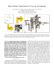### Adaptive Layout for Dynamically Aggregated Documents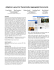### Slides.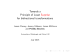### ATAC: A 1000-Core Cache-Coherent Processor with On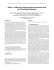### How to convert MTD Chipper/Shredder into Hammer Mill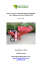### PubMed Advanced Guide - The Scripps Research Institute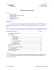### boilers controls - Building Services News### Optimizing Response Time, Rather than Hit Rates, of WWW Proxy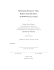### Volume 35, No. 4 April, 2006 - Lionel Collectors Club of America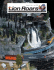### Nasal Polyposis: New Ways of Managing a Common Problem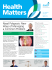### Securing concurrent programs with dynamic information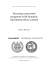### CTT 2007 Index - Classic Toy Trains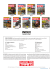### on the parallelization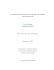### Colposcopy Standards - American Society for Colposcopy and